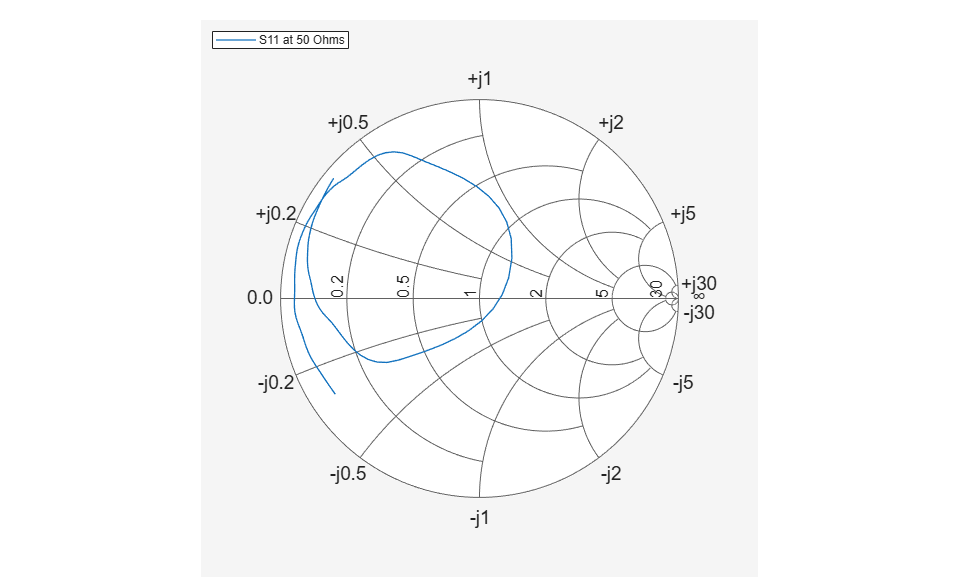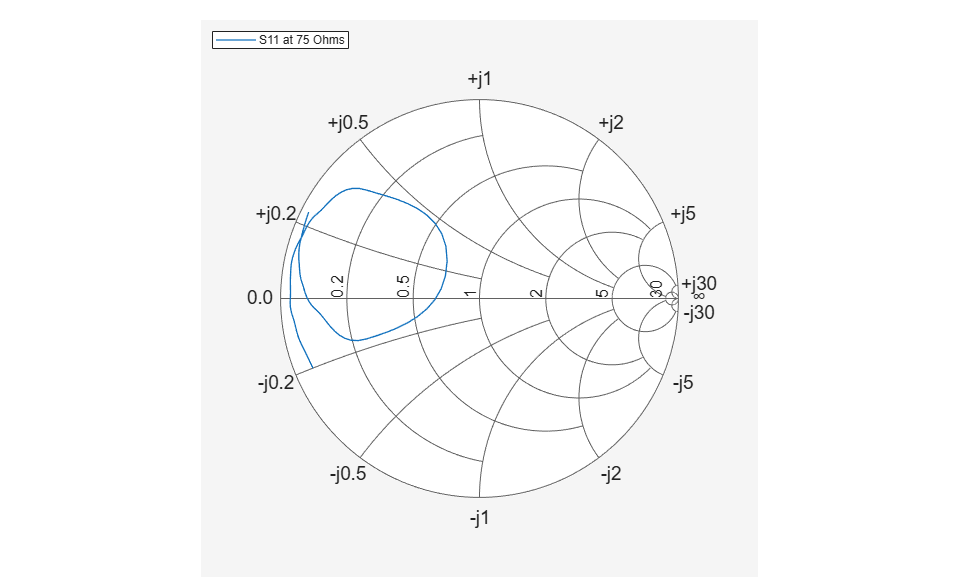# replace

Remove current data and add new data to Smith chart

## Syntax

``replace(plot,data)``
``replace(plot,frequency,data)``

## Description

example

````replace(plot,data)` removes all current data from a Smith chart, `plot`, and adds new data to the Smith chart.```
````replace(plot,frequency,data)` removes all current data and adds new data to the Smith chart based on multiple data sets containing frequencies corresponding to columns of the data matrix.```

## Examples

collapse all

```amp = read(rfckt.amplifier,'default.s2p'); Sa = sparameters(amp); smithplot(Sa,[1,1],'LegendLabels','S11 at 50 Ohms'); ```Plot S-parameter object with a new impedance of Z0 = 75 Ohms.

```Sa = sparameters(Sa,75); S11 = rfparam(Sa,1,1); Freq = Sa.Frequencies; s = smithplot('gco'); replace(s, Freq, S11); s.LegendLabels = 'S11 at 75 Ohms'; ```## Input Arguments

collapse all

Smith chart handle, specified as a plot handle. If the handle of the Smith chart is not retained during creation, use ```p = smithplot('gco')```.

Input data, specified as a complex vector or complex matrix.

For a matrix D, the columns of D are independent datasets. For N-by-D arrays, dimensions 2 and greater are independent datasets.

Data Types: `double`
Complex Number Support: Yes

Frequency data, specified as a real vector.

Data Types: `double`